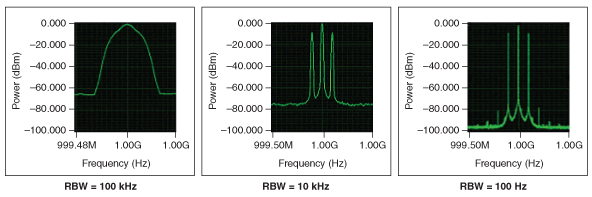이론 관련/전기 전자2021. 12. 22. 16:17

## FFT RBW - Resolution Band Width

FFT에서 얼마나 세밀하게 분석을 하냐의 단위

동일한 파형이라도 RBW가 낮아질수록 원본에 가까운 형태로 볼 수 있는 듯.

 The resolution bandwidth (RBW) determines the fast-Fourier transform (FFT) bin size, or the smallest frequency that can be resolved.+

2023.07.14

엇.. RBW 계산에 윈도우 타입에 따른 계수가 들어간다고..

kaiser window에서 beta1=16.7일 때 k=2.23 이라는데, k 값이 그러면 amplitude 관련 보정에 대한 그 계수가 아닌가?

 Where k is a window-related coeffcient, N is the number of time-domain samples used in the DFT calculation, and Fs is the sampling frequency. For the Kaiser window with beta1 = 16.7, k is about 2.23. The RBW shape factor, defned as the frequency ratio between the spectrum amplitude at 60 dB and 3 dB, is about 4:1. On the RSA5100/6100, the spectrum analysis measurement uses Equation 2 to calculate the required number of samples for the DFT based on the input span and RBW settings.

 15pRBW의 크기에 따라 측정되는 Noise의 크기도 달라짐. Dynamic Range 확보를 위해서는 상대적으로 작은 RBW가 요구된다.

+

2023.07.19

팔수록 이상한 용어만 잔뜩 나오냐 ㅠㅠ

RBW = (NENBW * samplerate) / input_length

인데 NENBW는 normalized effective noise bandwidth of the window 라..

 RBW - Resolution bandwidthResolution bandwidth of the estimate, returned as a scalar. The resolution bandwidth, RBW, is the smallest positive frequency, or frequency interval, that can be resolved. It is equal to NENBW*SampleRate/L, where L is the input length, and NENBW is the normalized effective noise bandwidth of the window. The data type of RBW matches the data type of the input.

equivalent noise bandwidth 라는 함수인데..

이건 또 어떻게 구현되는거냐.. normalized가 빠졌는데 동일한건가?

 bw = enbw(hamming(1000))bw = 1.3638bw = enbw(flattopwin(10000),44.1e3)bw = 16.6285

#### '이론 관련 > 전기 전자' 카테고리의 다른 글

ac dc coupling  (0) 2022.02.23 2022.02.22 2021.10.10 2021.10.09 2021.09.21